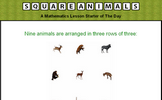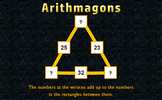# Equality and Equivalence

### Term 1 starting in week 5 :: Estimated time: 2 weeks

• Understand the meaning of equality
• Understand and use fact families, numerically and algebraically
• Solve one-step linear equations involving +/— using inverse operations
• Solve one-step linear equations involving x/÷ using inverse operations
• Understand the meaning of like and unlike terms
• Understand the meaning of equivalence
• Simplify algebraic expressions by collecting like terms, using the ? symbol

This page should remember your ticks from one visit to the next for a period of time. It does this by using Local Storage so the information is saved only on the computer you are working on right now.

## Lesson Starters

Here are some suggestions for whole-class, projectable resources which can be used at the beginnings of each lesson in this block.

### 1st Lesson#### Lost Sheep

Which algebraic expression is the odd one out?

### 2nd Lesson#### Mental Maths Quiz

A traditional twenty question mental arithmetic test presented as a PowerPoint presentation.

### 3rd Lesson#### Squared Animals

Separate three rows of three animals using three squares.

### 4th Lesson#### Tindice

How can you put the dice into the tins so that there is an odd number of dice in each tin?

### 5th Lesson#### Arithmagons

This lesson starter requires pupils to find the missing numbers in this partly completed arithmagon puzzle.

### 6th Lesson#### Ticker News

A Think Of A Number problem presented as a news ticker.

Some of the Starters above are to reinforce concepts learnt, others are to introduce new ideas while others are on unrelated topics designed for retrieval practice or and opportunity to develop problem-solving skills.

White Rose ResourcesEnd of block assessments provide a quick progress check at the end of each block of learning to make sure students have understood the content covered. This Scheme of Learning was produced by White Rose Maths and is used here with permission granted on 30th June 2021.For All: